Next: Equilibrium of Constant-Temperature System Up: Multi-Phase Systems Previous: Introduction

# Equilibrium of Isolated System

Consider a thermally isolated system,. According to Section 5.6, any spontaneously occurring process is such that the system's entropy tends to increase in time. In statistical terms, this means that the system evolves toward a situation of greater intrinsic probability. Thus, in any spontaneous process, the change in entropy satisfies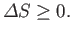(9.1)

It follows that if a stable equilibrium state has been attained [i.e., one in which no further spontaneous processes (other than random fluctuations) can take place] then this state is such that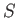is maximized. In other words, it is the most probable state of the system, subject to the given constraints. Thus, we can make the following statement:
For a thermally isolated system, the stable equilibrium state is such that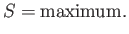Now, in a thermally isolated system, the first law of thermodynamics implies that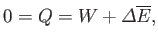(9.2)

or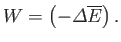(9.3)

If the external parameters of the system (e.g., its volume) are kept fixed, so that no work is done (i.e.,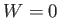), then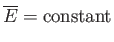(9.4)

asevolves toward its maximum value.

We can phrase the previous argument in more explicit statistical terms. Suppose that an isolated system is described by a parameter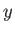(or by several such parameters), but that its total energy is constant. Let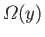denote the number of microstates accessible to the system when this parameter lies betweenand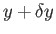(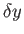being some fixed small interval). The corresponding entropy of the system is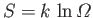. (See Section 5.6.) If the parameteris free to vary then the principle of equal a priori probabilities asserts that, in an equilibrium state, the probability,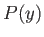, of finding the system with the parameter betweenandis given by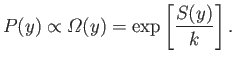(9.5)

(See Section 3.3.) The previous expression shows explicitly that the most probable state is one in whichattains a maximum value, and also allows us to determine the relative probability of fluctuations about this state.Next: Equilibrium of Constant-Temperature System Up: Multi-Phase Systems Previous: Introduction
Richard Fitzpatrick 2016-01-25